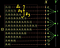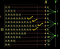# The function of a random variable

The random variable itself is a function and we are interested in the function of the random variable so it's essentially the function of a function.

Let’s say we have a function ‘’, then we can define a new function on top of it say cosine(x²) so here also we have the function of a function.

It’s important in the case of a random variable as the function of a random variable itself is a random variable. The outcome of an experiment is going to be random and since random variable maps the outcome to some values, the output from a random variable is going to be random and hence the value that the function of a random variable can take is going to be random.

Here is an example, let’s say we have a random variable ‘X’ which maps the outcomes of rolling two dice to the sum of two numbers

Let’s say we have another random variable ‘Y’ which is a function of random variable ‘X

The way random variable ‘Y’ works is that if the sum(of the numbers of two dice, output from the random variable ‘X’) is less than 5, then it maps to say 1 point if the sum is from 5 to 8, then Y maps to 2 points, and so on.

We are interested in the expected value of this random variable ‘Y

There are two ways to compute the expected value of the random variable ‘Y’: one would be to compute the distribution of ‘Y’ i.e the probability of each value that the random variable ‘Y’ can take and we can then use the standard formula to compute the expected value of ‘Y’. The other way would be to use the distribution of the random variable ‘X’ and since ‘Y’ is a function of ‘X’, we leverage the distribution of ‘X’ and compute the expected value of ‘Y’ using that.

Let’s compute the expected value using the first approach:

It’s the weighted sum of the values that the random variable ‘Y’ can take wherein the weights corresponds to the probabilities of corresponding values

pᵧ(1) is the probability that the random variable ‘Y’ can take on the value 1

Now the random variable ‘Y’ can have the value as 1 if the random variable ‘X’ can take on values as 2, or 3, or 4 and ‘X’ can take on either of these values when we have the corresponding outcomes as { (1,1), (1,2), (2,1), (1,3), (2,2), (3,1) } or we can call it as the union of three disjoint eventsThe case that X = 8 maps to Y as 2.

And we can compute the probability that the random variable ‘Y’ takes on the value as 1 as below:

‘1/36’ is the probability of A₁

‘2/36’ is the probability of A₂

‘3/36’ is the probability of A₃

Similarly, we can compute the probability of the case that the random variable ‘Y’ takes on the value 2, in this case, the marked 4 events in the below image are the outcomes of interestThe case that X = 8 maps to Y as 2.

Similarly, we have the probability that the random variable ‘Y’ can take the value as 3 as the following:

Let’s compute the same thing using the other approach:

We already know the distribution of ‘X’ but we ignore it in the first approach and compute the distribution of ‘Y

We notice that there is a mapping between ‘X’ and ‘Y’ for example ‘Y’ being 1 equals the scenario that X equals 2 union X equals 3 union X equals 4 and the probability of these are already known in the distribution of ‘X

Because ‘Y’ is a function of ‘X’, there is already a relationship between the distribution of ‘Y’ and the distribution of ‘X’.

Below is the expected value of ‘Y’ as from the first approach

We can open up the brackets and the above result can be written as:

Now we already got an expression for the expected value of ‘Y’ in terms of the probability of random variable ‘X’ taking on different values and interesting thing to note is that the probabilities of all the terms/values that the random variable ‘X’ can take are included in this formula

We can write this as below:

where the function g() corresponds to the value of ‘Y’ corresponding to the input to pₓ() for example when we are computing pₓ(2) we are interested in the probability of the random variable ‘X’ taking on the value 2, this quantity is then multiplied by g(2) which would be the value of ‘Y’ for input/x as 2.

And we can write the formula compactly as below:

It says that to compute the expectation of ‘Y’, sum over all the values that the random variable can take, take probabilities of those values of ‘X’ and multiply it with the value that the function g() would take for the respective value of ‘X’.

So, this is a formula for the expectation of the random variable ‘Y’ which is a function of the random variable ‘X’ in terms of the distribution of ‘X’.

And both the approach gives the same result at the end.